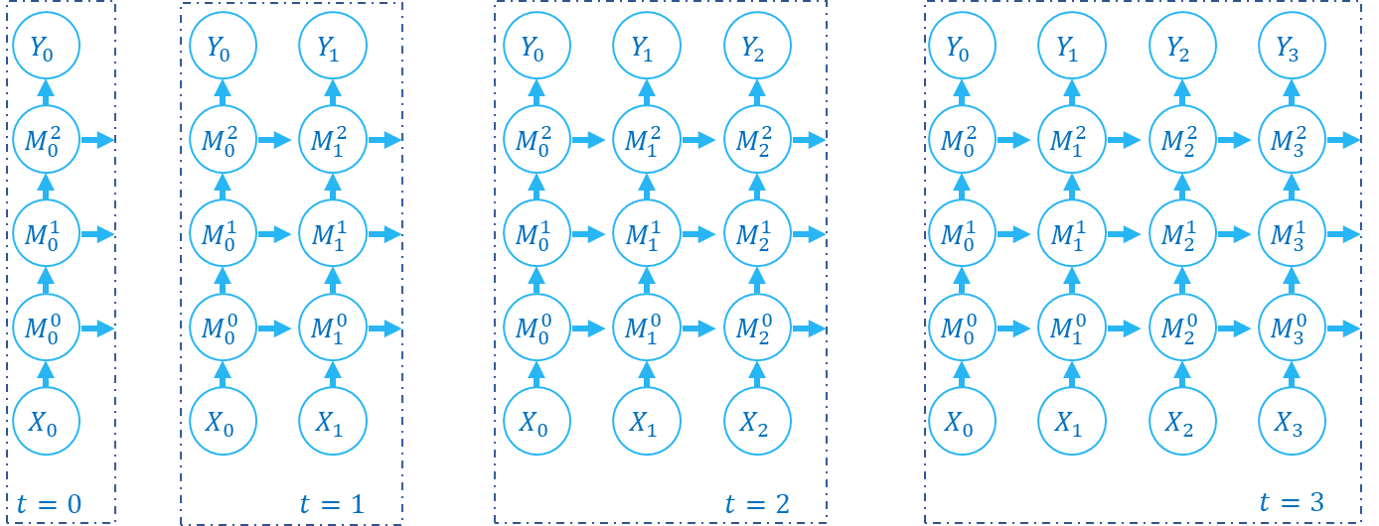# 传播模式¶

## 单步传播与多步传播¶

SpikingJelly中的绝大多数模块（spikingjelly.clock_driven.rnn 除外），例如 spikingjelly.clock_driven.layer.Dropout，模块名的前缀中没有 MultiStep，表示这个模块的 forward 函数定义的是单步的前向传播：

class MultiStepContainer(nn.Module):
def __init__(self, module: nn.Module):
super().__init__()
self.module = module

def forward(self, x_seq: torch.Tensor):
y_seq = []
for t in range(x_seq.shape):
y_seq.append(self.module(x_seq[t]))
y_seq[-1].unsqueeze_(0)

def reset(self):
if hasattr(self.module, 'reset'):
self.module.reset()


from spikingjelly.clock_driven import neuron, layer
import torch

neuron_num = 4
T = 8
if_node = neuron.IFNode()
x = torch.rand([T, neuron_num]) * 2
for t in range(T):
print(f'if_node output spikes at t={t}', if_node(x[t]))
if_node.reset()

ms_if_node = layer.MultiStepContainer(if_node)
print("multi step if_node output spikes\n", ms_if_node(x))
ms_if_node.reset()


if_node output spikes at t=0 tensor([1., 1., 1., 0.])
if_node output spikes at t=1 tensor([0., 0., 0., 1.])
if_node output spikes at t=2 tensor([1., 1., 1., 1.])
if_node output spikes at t=3 tensor([0., 0., 1., 0.])
if_node output spikes at t=4 tensor([1., 1., 1., 1.])
if_node output spikes at t=5 tensor([1., 0., 0., 0.])
if_node output spikes at t=6 tensor([1., 0., 1., 1.])
if_node output spikes at t=7 tensor([1., 1., 1., 0.])
multi step if_node output spikes
tensor([[1., 1., 1., 0.],
[0., 0., 0., 1.],
[1., 1., 1., 1.],
[0., 0., 1., 0.],
[1., 1., 1., 1.],
[1., 0., 0., 0.],
[1., 0., 1., 1.],
[1., 1., 1., 0.]])


## 逐步传播与逐层传播¶

if_node = neuron.IFNode()
x = torch.rand([T, neuron_num]) * 2
for t in range(T):
print(f'if_node output spikes at t={t}', if_node(x[t]))


net = nn.Sequential(M0, M1, M2)

for t in range(T):
Y[t] = net(X[t])net = nn.Sequential(M0, M1, M2)

Y = net(X)for t in range(T):
y[t] = fc(x[t])  # x.shape=[T, batch_size, in_features]


y = fc(x)  # x.shape=[T, batch_size, in_features]


with torch.no_grad():
T = 16
batch_size = 8
x = torch.rand([T, batch_size, 4])
fc = SeqToANNContainer(nn.Linear(4, 2), nn.Linear(2, 3))
print(fc(x).shape)


torch.Size([16, 8, 3])